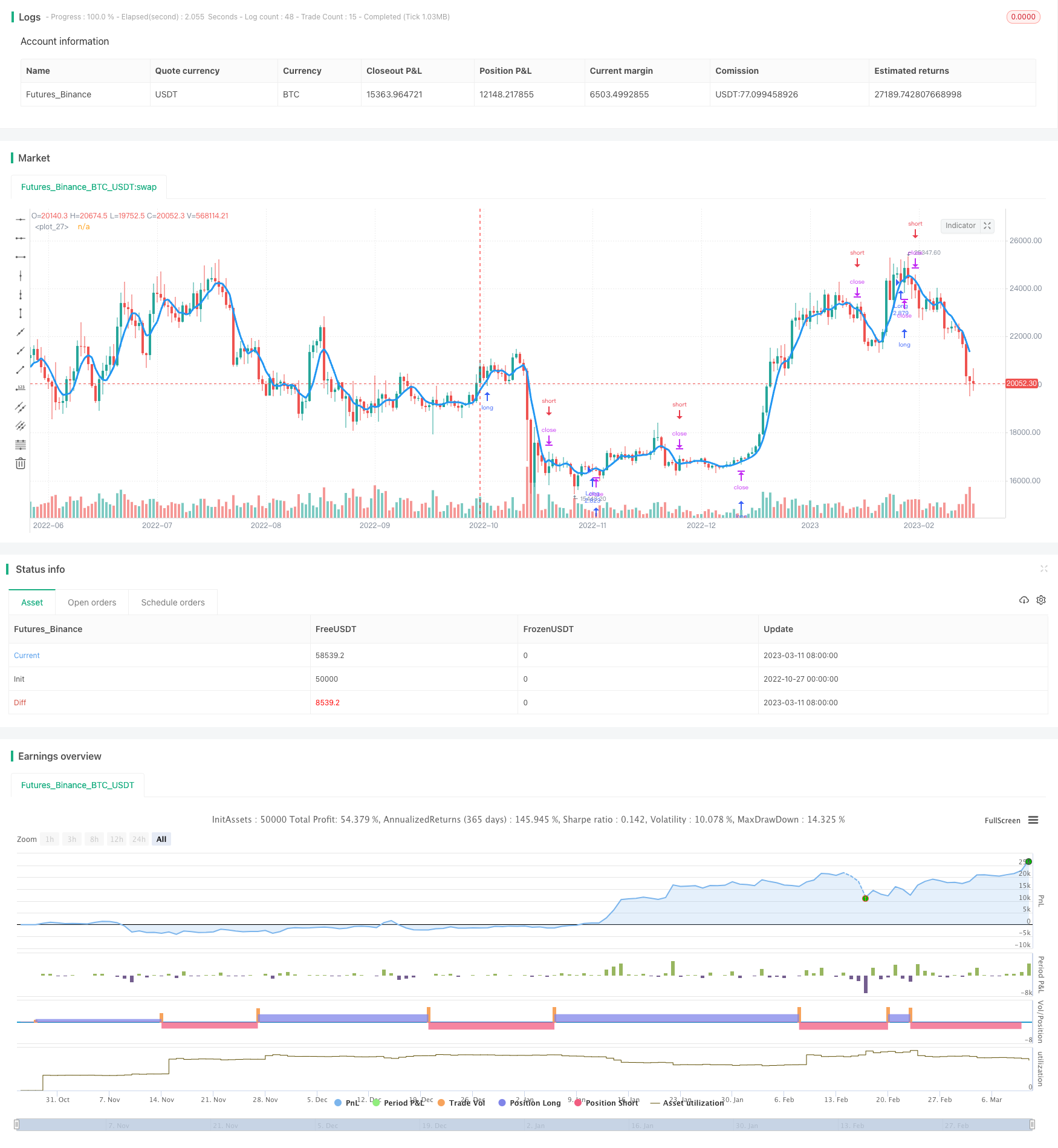# Cross Limit Order Cross Period Trading Strategy

Author: ChaoZhang, Date: 2023-11-03 17:11:34
Tags:### Summary

This strategy is a trend following trading strategy that uses simple moving average to determine market trend direction and place limit orders along the moving average line to follow the trend.

### Strategy Logic

1. Calculate the Simple Moving Average (SMA) and the trend direction trend.

2. If Anti-Saw filter is enabled, uptrend is identified when lows are above SMA, downtrend is identified when highs are below SMA. If Anti-Saw filter is disabled, uptrend is identified when close is above SMA, downtrend is identified when close is below SMA.

3. Place limit orders at SMA price according to the trend direction trend and enabled trading directions needlong and needshort:

• If long trade is needed (needlong is true) and in uptrend, place long limit order at SMA price

• If short trade is needed (needshort is true) and in downtrend, place short limit order at SMA price

4. Set stop loss logic to exit positions if position direction does not match trend direction.

5. Only trade within specified date range based on date range parameters.

1. Using SMA to determine trend can effectively filter market noise and lock in longer term trend.

2. Placing limit orders at SMA price can get good entry points when trend starts.

3. Flexibility to only go long or short according to personal trading style.

4. Stop loss in place to avoid enlarging losses.

5. Trading time range sets to avoid volatility around major events.

### Risk Analysis

1. SMA as trend indicator has lagging effect, may miss trend turning points and cause losses.

2. Limit orders lack flexibility, may not get into positions due to short term trend adjustments.

3. SMA period parameter needs proper configuration, improper settings lead to wrong trend determination.

4. Trading session parameters need to be reasonable to avoid missing opportunities or trading in risky periods.

### Optimization Directions

1. Consider adding other indicators for multi-indicator confirmation, avoiding SMA lagging issues.

2. Switch to market order trailing when price breaks SMA, improving tracking flexibility.

3. Dynamically optimize SMA period to adapt to different market cycles.

4. Set stop loss to swing low/high instead of strictly at SMA price for more flexible stops.

5. Increase algorithmic elements for smarter dynamic trading sessions avoiding major risk events.

### Summary

Overall this is a relatively simple trend following strategy, with the core idea of determining trend direction with SMA and placing limit orders at SMA price to follow the trend. With certain optimizations it can improve flexibility, adaptability and intelligence. The strategy is easy to understand and implement, suitable for algorithmic trading beginners, but requires risk awareness, cautious backtest evaluation, strict monitoring and optimization for live trading.

```/*backtest
start: 2022-10-27 00:00:00
end: 2023-03-12 00:00:00
period: 1d
basePeriod: 1h
exchanges: [{"eid":"Futures_Binance","currency":"BTC_USDT"}]
*/

//Noro
//2020

//@version=4
strategy(title = "Noro's CrossLimit", shorttitle = "CrossLimit", overlay = true, default_qty_type = strategy.percent_of_equity, default_qty_value = 100.0, pyramiding = 0, commission_value = 0.0)

needlong = input(true, "long")
needshort = input(true, "short")
lotsize = input(100, defval = 100, minval = 1, maxval = 10000, title = "Lot, %")
src = input(close, defval = close, title = "MA Source")
len = input(5, defval = 5, minval = 1, title = "SMA length")
off = input(0, defval = 0, minval = 0, title = "SMA offset")
anti = input(true, defval = true, title = "Anti-saw filter")
rev = input(false, defval = false, title = "Reverse")
showma = input(true, defval = true, title = "Show MA")
showbg = input(false, defval = false, title = "Show background")
fromyear = input(1900, defval = 1900, minval = 1900, maxval = 2100, title = "From Year")
toyear = input(2100, defval = 2100, minval = 1900, maxval = 2100, title = "To Year")
frommonth = input(01, defval = 01, minval = 01, maxval = 12, title = "From Month")
tomonth = input(12, defval = 12, minval = 01, maxval = 12, title = "To Month")
fromday = input(01, defval = 01, minval = 01, maxval = 31, title = "From day")
today = input(31, defval = 31, minval = 01, maxval = 31, title = "To day")

//MA
ma = sma(src, len)[off]
macol = showma ? color.blue : na
plot(ma, color = macol, linewidth = 3, transp = 0)

//Background
trend = 0
trend := anti == false and close > ma ? 1 : anti == false and close < ma ? -1 : low > ma ? 1 : high < ma ? -1 : trend
bgcol = showbg ? trend == 1 ? color.lime : trend == -1 ? color.red : na : na
bgcolor(bgcol, transp = 70)

//Signals
bar = close > open ? 1 : close < open ? -1 : 0
up = (trend == 1 and rev == false) or (trend == -1 and rev == true)
dn = (trend == -1 and rev == false) or (trend == 1 and rev == true)

size = strategy.position_size
truetime = time > timestamp(fromyear, frommonth, fromday, 00, 00) and time < timestamp(toyear, tomonth, today, 23, 59)
lot = 0.0
lot := size != size ? strategy.equity / close * lotsize / 100 : lot
if trend != 0
strategy.entry("Long", strategy.long, lot, limit = ma, when = needlong and truetime and up)
strategy.entry("Short", strategy.short, lot, limit = ma, when = needshort and truetime and dn)
if size > 0 and needshort == false and trend == -1
strategy.exit("Stop Long", "Long", limit = ma)
if size < 0 and needlong == false and trend == 1
strategy.exit("Stop Short", "Short", limit = ma)
if time > timestamp(toyear, tomonth, today, 23, 59)
strategy.close_all()
strategy.cancel("Long")
strategy.cancel("Short")
```

More